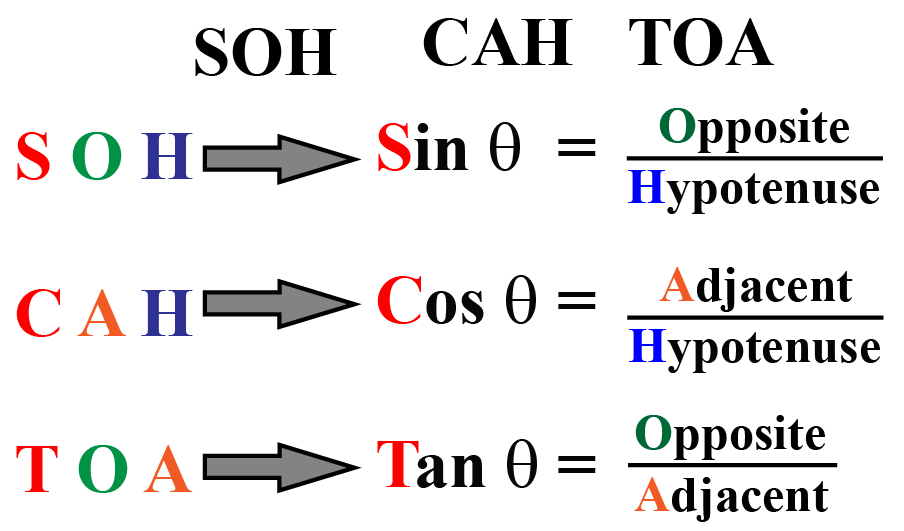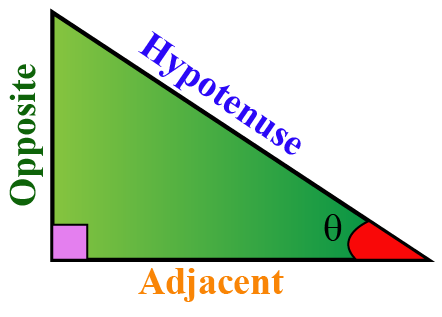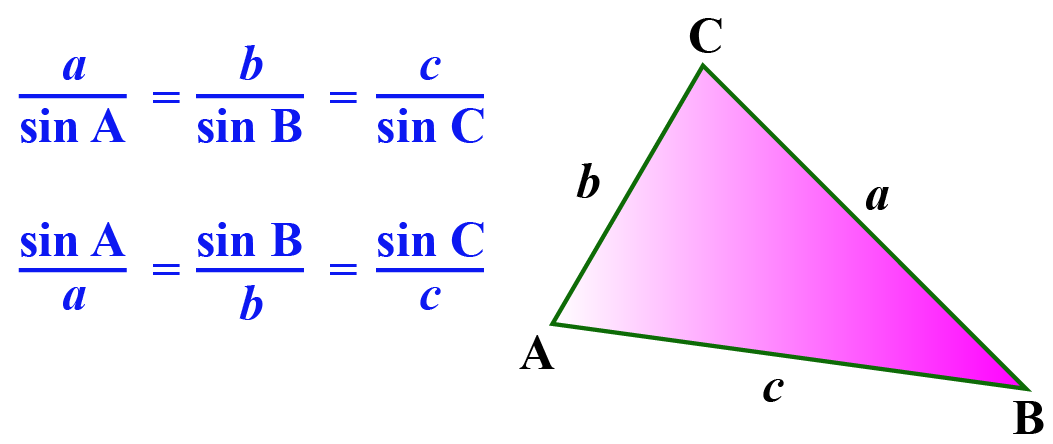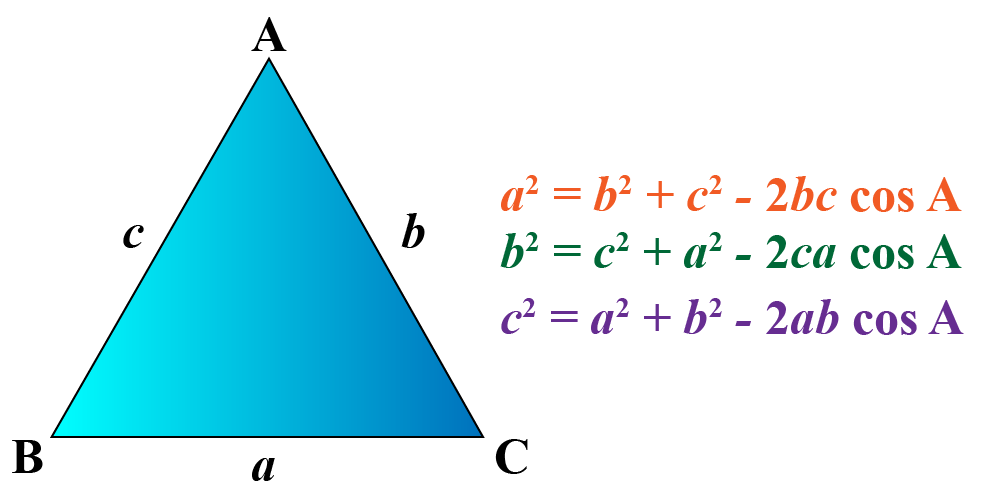# Trigonometric Identities

Trigonometric Identities
Go back to  'Trigonometry'

In this mini-lesson, we will explore trigonometric identities.

We have already learned the primary trigonometric ratios sin, cos, and tan.We have also learned that there are three other trigonometric ratios sec, csc, and cot which are the reciprocal of sin, cos, and tan respectively.

How are these trigonometric ratios connected with each other?

They are connected through trigonometric identities (or trig identities).

Let's start learning about trigonometric identities.

## What Are Trig Identities?

Trigonometric identities are equations that relate different trigonometric functions and are true for any value of the variable that is there in the domain.

Basically, an identity is an equation that holds true for all the values of the variable(s) present in it.

For example, some of the algebraic identities are:

$\begin{array}{l} (a+b)^{2}=a^{2}+2 a b+b^{2} \\ (a-b)^{2}=a^{2}-2 a b+b^{2} \\ (a+b)(a-b)=a^{2}-b^{2} \end{array}$

The algebraic identities relate just the variables whereas the trig identities relate the 6 trigonometric functions sin, cos, tan, csc, sec, and cot.

You can explore different types of trigonometric identities using the following simulation.

Let's learn about each type of identities in detail.

## Reciprocal Identity

We already know that the reciprocals of sin, cos, and tan are csc, sec, and cot respectively.

Thus, the reciprocal identities are:

 Reciprocal Identities \begin{align} \sin \theta &= \dfrac{1}{\csc \theta} \text{ (OR) } \csc \theta= \dfrac{1}{ \sin \theta}\\[0.2cm] \cos\theta &= \dfrac{1}{\sec \theta} \text{ (OR) } \sec\theta= \dfrac{1}{ \cos\theta}\\[0.2cm] \tan\theta &= \dfrac{1}{\cot\theta} \text{ (OR) } \cot\theta= \dfrac{1}{ \tan\theta} \end{align}

## Pythagorean Identities

The Pythagorean identities in trigonometry are derived from Pythagoras theorem.

Applying Pythagoras theorem to the right-angled triangle below, we get:\begin{align} \text{Opposite}^2+ \text{Adjacent}^2&= \text{Hypotenuse}^2\\[0.2cm] \text{Dividing both sides by }&\text{Hypotenuse}^2\\[0.2cm] \dfrac{ \text{Opposite}^2}{\text{Hypotenuse}^2}+ &\dfrac{ \text{Adjacent}^2}{\text{Hypotenuse}^2}=1\\[0.2cm] \sin^2 \theta+& \cos^2\theta=1 \end{align}

This is one of the Pythagorean identities. In the same way, we can derive two other Pythagorean identities.

 Pythagorean Identities $\begin{array}{l} \sin ^{2} \theta+\cos ^{2} \theta=1 \\ 1+\tan ^{2} \theta=\sec ^{2} \theta \\ 1+\cot ^{2} \theta=\csc ^{2} \theta \end{array}$

## Complement and Supplement of a Ratio

The complement of an angle $$\theta$$ is $$90-\theta$$.

The trigonometric ratios of complementary angles are:

 $\begin{array}{l|l} \sin \left(90^{\circ}-\theta\right)=\cos \theta \\ \cos \left(90^{\circ}-\theta\right)=\sin \theta \\ \csc \left(90^{\circ}-\theta\right)=\sec \theta \\ \sec \left(90^{\circ}-\theta\right)=\csc \theta\\ \tan \left(90^{\circ}-\theta\right)=\cot \theta \\ \cot \left(90^{\circ}-\theta\right)=\tan \theta \end{array}$

The supplement of an angle $$\theta$$ is $$180-\theta$$.

The trigonometric ratios of supplementary angles are:

 $\begin{array}{l|l} \sin \left(180^{\circ}-\theta\right)=\sin\theta \\ \cos \left(180^{\circ}-\theta\right)=-\cos \theta \\ \csc \left(180^{\circ}-\theta\right)=\csc \theta \\ \sec \left(180^{\circ}-\theta\right)=-\sec \theta\\ \tan \left(180^{\circ}-\theta\right)=-\tan \theta \\ \cot \left(180^{\circ}-\theta\right)=-\cot \theta \end{array}$

## Sum and Difference Formulas

The sum and difference identities include the formulas of sin(a+b), cos(a-b), cot(a+b), etc.

 Sum and Difference Identities $\begin{array}{l} \sin (A\:+B)=\sin A \cos B\:+\cos A \sin B \\[0.2cm] \sin (A\:-B)=\sin A \cos B\:-\cos A \sin B \\[0.2cm] \cos (A\:+B)=\cos A \cos B\:-\sin A \sin B \\[0.2cm] \cos (A\:-B)=\cos A \cos B\:+\sin A \sin B \\[0.2cm] \tan (A\:+B)=\dfrac{\tan A\:+\tan B}{1\:-\tan A \tan B} \\[0.2cm] \tan (A\:-B)=\dfrac{\tan A\:-\tan B}{1\:+\tan A \tan B} \end{array}$

## Double Angle Formulas

The double angle identities can be obtained by using the sum and difference formulas.

For example, from the above formulas:

$\sin (A+B)=\sin A \cos B+\cos A \sin B$

Substitute $$A=B=\theta$$ on both sides here, we get:

\begin{align}\sin (\theta +\theta )&=\sin \theta \cos \theta +\cos \theta \sin \theta \\[0.2cm] \sin 2\theta &= 2 \sin \theta \cos \theta \end{align}

In the same way, we can derive the other double angle identities.

 Double Angle Identities \begin{aligned} \sin 2 \theta &=2 \sin \theta \cos \theta \\[0.2cm] \cos 2 \theta &=\cos ^{2} \theta-\sin ^{2} \theta \\ &=2 \cos ^{2} \theta-1 \\ &=1-2 \sin ^{2} \theta \\[0.2cm] \tan 2 \theta &=\frac{2 \tan \theta}{1-\tan ^{2} \theta} \end{aligned}

## Half Angle Formulas

Using one of the above double angle formulas,

\begin{align} \cos 2\theta &= 1-2 \sin ^{2} \theta\\[0.2cm] 2 \sin^2\theta &= 1- \cos 2\theta\\[0.2cm] \sin^2\theta&= \dfrac{1-\cos2\theta}{2}\\[0.2cm] \sin \theta&=\pm \sqrt{\dfrac{1-\cos 2\theta}{2}} \end{align}

Replacing $$\theta$$ by $$\dfrac{\theta}{2}$$ on both sides,

$\sin \frac{\theta}{2}=\pm \sqrt{\frac{1-\cos \theta}{2}}$

This is the half-angle formula of sin.

In the same way, we can derive the other half-angle formulas.

 Half-Angle Formulas $\begin{array}{l} \sin \dfrac{\theta}{2}=\pm \sqrt{\dfrac{1-\cos \theta}{2}} \\[0.2cm] \cos \dfrac{\theta}{2}=\pm \sqrt{\dfrac{1+\cos \theta}{2}} \\[0.2cm] \tan \dfrac{\theta}{2}=\pm \sqrt{\dfrac{1-\cos \theta}{1+\cos \theta}} \end{array}$Tips and Tricks
1. To write the trigonometric ratios of complementary angles, we consider the following as pairs: (sin, cos), (csc, sec), and (tan, cot).
2. While writing the trigonometric ratios of supplementary angles, the trigonometric ratio won't change. The sign can be decided using the fact that only sin and csc are positive in the second quadrant where the angle is of the form $$180-\theta$$.
3. There are 3 formulas for cos 2x formula. Among them, you can remember just the first one because the other two can be obtained by the Pythagorean identity $$\sin^2 x+\cos^2x=1$$.
4. The half-angle formula of tan is obtained by applying the identity tan = sin/cos and then using the half-angle formulas of sin and cos.

The trigonometric identities that we have learned are derived using the right-angled triangles.

There are a few other identities that we use in the case of the triangles that are not right-angled.

### Sine Rule

The sine rule gives the relation between the angles and the corresponding sides of a triangle.### Cosine Rule

The cosine rule gives the relation between the angles and the sides of a triangle and is usually used when two sides and the included angle of a triangle are given.## Solved Examples

 Example 1

Can we help Tina prove the following identity using the trig identities?

$$\frac{\sin ^{3} \theta+\cos ^{3} \theta}{\sin \theta+\cos \theta}+\sin \theta \cos \theta=1$$

Solution

We make use of the following identity:

$a^{3}+b^{3}=(a+b)\left(a^{2}-a b+b^{2}\right)$

We use the Pythagorean identities to prove this identity.

\begin{align} &L H S\0.2cm] &=\frac{\sin ^{3} \theta+\cos ^{3} \theta}{\sin \theta+\cos \theta}+\sin \theta \cos \theta \\[0.2cm]&=\frac{(\sin \theta+\cos \theta)\left(\sin ^{2} \theta-\sin \theta \cos \theta+\cos ^{2} \theta\right)}{\sin \theta+\cos \theta}+\sin \theta \cos \theta \\[0.2cm] &=\left(\sin ^{2} \theta-\sin \theta \cos \theta+\cos ^{2} \theta\right)+\sin \theta \cos \theta \\[0.2cm] &=\sin ^{2} \theta+\cos ^{2} \theta\\[0.2cm]&=1\\[0.2cm]&=R H S \end{align}  Hence proved.  Example 2 Can we help Jake prove the following identity using the trig identities? $$(\sin \theta+\operatorname{cosec} \theta)^{2}+(\cos \theta+\sec \theta)^{2}=7+\tan ^{2} \theta+\cot ^{2} \theta$$ Solution We use the reciprocal identities and Pythagorean identities to prove this identity. \begin{align} &L H S\\[0.2cm]=& \sin ^{2} \theta+\operatorname{cosec}^{2} \theta+2\,\underbrace{ \sin \theta \operatorname{cosec} \theta}_{=1} \\[0.2cm] &+\cos ^{2} \theta+\sec ^{2} \theta+2\,\underbrace{ \cos \theta \sec \theta}_{=1} \\[0.2cm] =&\underbrace{\left(\sin ^{2} \theta+\cos ^{2} \theta\right)}_{=1}+ csc^2\theta + \sec^2\theta +2+2\\[0.2cm] =&1+\left(1+\cot ^{2} \theta\right) +\left(1+\tan ^{2} \theta\right)+2+2 \\[0.2cm] =& 7+\tan ^{2} \theta+\cot ^{2}\theta\\[0.2cm] =&R H S \end{align}  Hence proved.  Example 3 Can we help James find the exact value of $$\sin 75^\circ$$? Solution We know that \[75^\circ = 30^\circ+45^\circ

We apply the sum formula of sin to find the value of $$\sin 75^\circ$$.

$\sin (A+B) = \sin A \cos B +\cos A \sin B$

Substitute $$A=30^\circ$$ and $$B=45^\circ$$ here on both sides:

\begin{align} \sin (30^\circ + 45^\circ) &= \sin 30^\circ \cos 45^\circ+ \cos 30^\circ + \sin 45^\circ\0.2cm] \sin 75^\circ &= \dfrac{1}{2}\cdot \dfrac{\sqrt{2}}{2}+ \dfrac{\sqrt{3}}{2} \cdot \dfrac{\sqrt{2}}{2}\\[0.2cm] \sin 75^\circ&= \dfrac{\sqrt{2}+ \sqrt{6}}{4} \end{align} Here, the values of $$\sin 30^\circ, \cos 45^\circ, \cos 30^\circ$$ and $$\sin 45^\circ$$ can be obtained by using trigonometric table. Thus,  $$\sin 75^\circ= \dfrac{\sqrt{2}+ \sqrt{6}}{4}$$  Example 4 Given $$\cos \theta = \dfrac{3}{5}$$ where $$\theta$$ is an angle in the quadrant I, find the values of: a) $$\sin 2\theta$$ b) $$\sin \dfrac{\theta}{2}$$ Solution It is given that: \[\cos \theta = \dfrac{3}{5}

Using one of the Pythagorean identities:

\begin{align} \sin^2\theta+\cos^2\theta&=1\\[0.2cm] \sin^2\theta&= 1-\cos^2\theta\\[0.2cm] \sin \theta &= \pm\sqrt{1-cos^2\theta}\\[0.2cm] \sin \theta &= \pm \sqrt{1- \left( \dfrac{3}{5} \right)^2}\\[0.2cm] \sin \theta&= \pm \sqrt{\dfrac{16}{25}}\\[0.2cm] \sin \theta&= \pm \dfrac{4}{5} \end{align}

Since $$\theta$$ is in quadrant I, $$\sin \theta$$ is positive.

Thus, $\sin \theta= \dfrac{4}{5}$

a) Using the double angle formula of sin,

\begin{align} \sin 2 \theta &= 2 \sin \theta \cos \theta\\[0.2cm] &= 2 \left( \dfrac{4}{5}\right) \left(\dfrac{3}{5} \right)\\[0.2cm] &= \dfrac{24}{25} \end{align}

b) Using the half-angle formula of sin,

\begin{align} \sin \dfrac{ \theta}{2} &= \pm \sqrt{\frac{1-\cos \theta}{2}}\\[0.2cm] &= \pm \sqrt{\frac{1- \dfrac{3}{5}}{2}}\\[0.2cm] &= \pm \dfrac{1}{\sqrt{ 5}}\\[0.2cm] &= \dfrac{\sqrt{5}}{5} \end{align}

Thus:

 \begin{align} \sin 2 \theta &= \dfrac{24}{25}\\[0.2cm] \sin \dfrac{ \theta}{2} &= \dfrac{\sqrt{5}}{5} \end{align}Challenging Questions
1. Can you help Sophia prove the following identity?
$$\frac{\sin \theta-\cos \theta+1}{\sin \theta+\cos \theta-1}=\frac{1}{\sec \theta-\tan \theta}$$
2. Find the value of $$\sin 22 \dfrac 1 2^\circ$$.

## Interactive Questions

Here are a few activities for you to practice.

Select/type your answer and click the "Check Answer" button to see the result.

## Let's Summarize

The mini-lesson targeted the fascinating concept of Trigonometric Identities. The math journey around Trigonometric Identities starts with what a student already knows, and goes on to creatively crafting a fresh concept in the young minds. Done in a way that not only it is relatable and easy to grasp, but also will stay with them forever. Here lies the magic with Cuemath.

## About Cuemath

At Cuemath, our team of math experts is dedicated to making learning fun for our favorite readers, the students!

Through an interactive and engaging learning-teaching-learning approach, the teachers explore all angles of a topic.

Be it worksheets, online classes, doubt sessions, or any other form of relation, it’s the logical thinking and smart learning approach that we, at Cuemath, believe in.

## 1. How to memorize trig identities?

Try to derive the trigonometric identities using the procedures that are explained on this page.

If you can derive them on your own, then memorizing them will not be a problem.

## 2. What trig identities we must know definitely?

All trig identities are used in solving the problems. The main trig identities are Pythagorean identities, reciprocal identities, sum and difference identities, double angle and half-angle identities.

For the non-right-angled triangles, we will have to use the sine rule and the cosine rule.

## 3.  When do you use the double angle formula?

The double angle formulas are used when the angle is of the form 2 multiplied by a variable.

Download Trigonometry Worksheets
Trigonometry
Grade 10 | Answers Set 1
Trigonometry
Grade 9 | Questions Set 1
Trigonometry
Grade 9 | Answers Set 1
Trigonometry
Grade 10 | Questions Set 1

More Important Topics
Numbers
Algebra
Geometry
Measurement
Money
Data
Trigonometry
Calculus